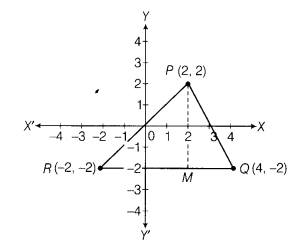# Plot the points (2,2), (4,-2) and (-2,-2) and check whether they are collinear or not

Plot the points (2,2), (4,-2) and (-2,-2) and check whether they are collinear or not.

Plotting the points on the graph, we get the points P(2,2), Q(4, -2) and R(-2, -2). On joining these three points, we get three lines, i.e. the given points do not lie on the same line. So, given points are not collinear.